# NaCaX

Model number
0055

An ion channel, a sodium calcium exchanger in the surface membrane (sarcolemma) of mammalian pacemaker cells. A mathematical model for the electrophysiological responses.

## Detailed Description

A sodium calcium exchange model. Sodium calcium ion channels exist in the surface membrane (sarcolemma) of a muscle cell. At allows sodium ions to flow down the electrochemical gradient of sodium, across the plasma membrane in exchange for the countertransport of calcium ions. Thus, the maintenance of calcium contentration in cells can be maintained. In this model, it is in regard to the maintenance of calcium concentration in the sarcoplasmic reticulum of cardiac cells. This model is derived from the Demir, Clark, Murphey, and Giles model: A mathematical model of a rabbit sinoatrial node cell. The Demir et al model is a mathematical model for the electrophysiological responses of a rabbit sinoatrial node cell. It describes many ion channels causing known currents in mammalian pacemaker cells. The sodium calcium exchanger is focused on for this model.

## Figures

The following figures were all generated with JSim. They can be reproduced by downloading the project file, or running the applet (below).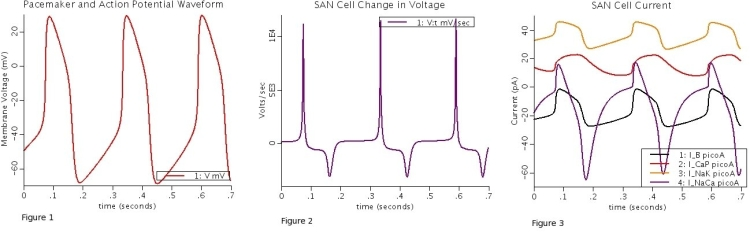Figure 1 displays the pacemaker and action potential waveforms. Figure 2 shows the first derivative (dV/dt) of the model-generated waveform. Figure three gives the total background (IB), pump (INaK and ICaP), and exchanger (INaCa) currents.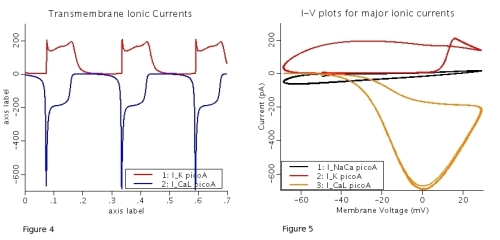Figure 4 displays the transmembrane ionic currents (ICa,L and IK). Figure 5 gives the Current vs Voltage plots for the major ionic currents (ICa,L, IK, and INaCa).

All currents are given in picoamps, all voltages are in millivolts, and all time is given in seconds.

## Relevant Equations

A complete list of equations can be found in the source code of the project file. The following equations are for only the pump and exchanger currents, as well as the differential equation describing the membrane potential (voltage).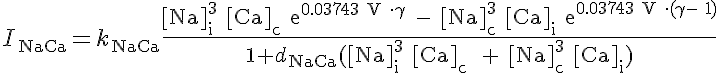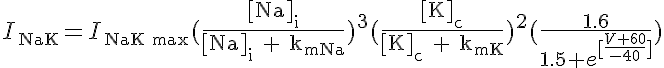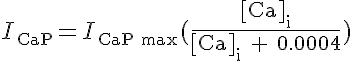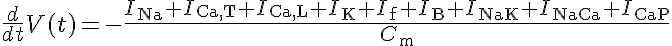References

S.S. Demir, J.W. Clark, C.R. Murphey, W.R. Giles, A mathematical model of a rabbit sinoatrial node cell, Modeling in Physiology, American Physiological Society C832 - C852, 1994

Key terms
Sodium calcium exchanger
ion channel
sarcolemma
sarcoplasmic reticulum
cardiac cell
sinoatrial